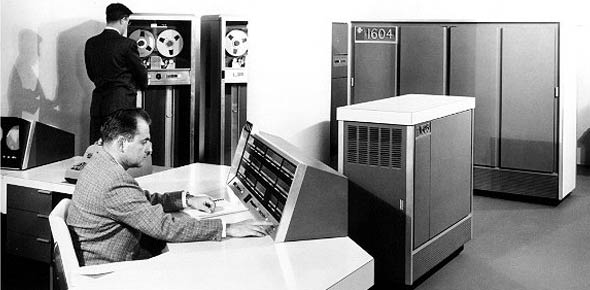# Vol3 CDC 3D153 Ure2

21 Questions | Total Attempts: 156SettingsThis is to be used as a study aid for the 3D153 CDC's.

• 1.
(403) The closest point of orbit that a satellite comes to the Earth is called the?
• A.

Apogee

• B.

Perigee

• C.

Major axis

• D.

Minor axis

• 2.
(403) What is the inclination angle of a polar orbit?
• A.

0 degrees

• B.

45 degrees

• C.

90 degrees

• D.

180 degrees

• 3.
(404) What are the two types of communications satellites?
• A.

Active and Passive

• B.

Active and Real-time

• C.

Delay and Real-time

• D.

Delay and Passive

• 4.
(404) Which satellite reflects signals transmitted toward it?
• A.

Active

• B.

Delay

• C.

Passive

• D.

Real-time

• 5.
(404) An advantage of passive satellites is that they
• A.

Have a larger transmitter power

• B.

Have more sensitive equipment.

• C.

Operate as a repeater station in space

• D.

Operate over a wider-frequency range.

• 6.
What is the time delay of a real-time satellite?
• A.

0.1 seconds

• B.

0.6 seconds

• C.

1 second

• D.

6 seconds

• 7.
(405) What satellite access technique was the first multiple access method developed?
• A.

Code division multiple access (CDMA).

• B.

Time division multiple access (TDMA).

• C.

Demand assigned multiple access (DAMA).

• D.

Frequency Division Multiple Access (FDMA)

• 8.
(405) What satellite access technique is being used when all channels are pooled and used by any station, according to its instantaneous traffic load?
• A.

Code division multiple access (CDMA)

• B.

Time division multiple access (TDMA)

• C.

Demand assigned multiple access (DAMA)

• D.

Frequency Division multiple access (FDMA)

• 9.
(406) When computing satellite look angles, ground distance on the earth’s surface is expressed in?
• A.

Degrees

• B.

Seconds

• C.

Minutes

• D.

Nautical miles

• 10.
(406) One degree of arc on the Earth’s surface equals
• A.

One statute mile

• B.

One nautical mile

• C.

69 statute miles

• D.

69 nautical miles

• 11.
(407) Solar time is based on?
• A.

Atomic clock oscillators

• B.

Apparent constellation movement.

• C.

The moon's rotation around the sun

• D.

The apparent motion of the sun in the sky

• 12.
(407) An atomic time scale is based on the frequency of which type of oscillator?
• A.

Pendulum

• B.

Crystal

• C.

Quartz

• D.

Cesium

• 13.
(408) On what kind of clock system is coordinated universal time based?
• A.

Amplitude modulation and pulse modulation

• B.

Daylight savings

• C.

24-hour

• D.

12-hour

• 14.
(409) The international agency responsible for coordinated universal time (UTC) is the?
• A.

United States Naval Observatory

• B.

International Bureau of Weights and Measures.

• C.

Precision Measurement Equipment Laboratory.

• D.

National Institute of Standards and Technology.

• 15.
Uploading satellite control data, tracking ballistic missiles, and predicting satellite tracking coordinates are examples of missions where all parties involved must have?
• A.

Identical time code formats.

• B.

Different time code formats.

• C.

Synchronized timing

• D.

Offset timing.

• 16.
(410) What two factors cause a quartz oscillator’s resonant frequency to drift?
• A.

Humidity and aging.

• B.

Temperature and aging.

• C.

Humidity and operating frequency.

• D.

Temperature and operating frequency.

• 17.
(410) The operation of the atomic clock is based on the phenomenon that
• A.

Crystals resonate when an electric charge is applied.

• B.

Atoms release energy at a particular resonant frequency.

• C.

Atoms are sensitive to temperature and directional effects.

• D.

When atoms are split they produce predictable nuclear reactions.

• 18.
(411) Which type of modems transmits RF signals over coaxial cable?
• A.

Voice

• B.

Cable

• C.

• D.

Wireless

• 19.
What is multiplexing?
• A.

Transmitting one signal over multiple transmission paths

• B.

Transmitting one signal multiple times over a single transmission path.

• C.

Combining multiple signals for transmission over a single transmission path.

• D.

Combining multiple signals for transmission over separate, individual transmission paths.

• 20.
(412) What technique of multiplexing samples each low speed channel in sequence to interleave bits or characters and then transmit them at high speed?
• A.

Wavelength division

• B.

Frequency division

• C.

Time division

• D.

Code division

• 21.
(412) Wavelength division multiplexing assigns each incoming optical signal to a specific?
• A.

Pseudorandom code.

• B.

Frequency of light.

• C.Back to top